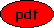On the Numerical Analysis and Visualisation of Implicit Ordinary Differential Equations Co-author(s): Elishan Braun and Matthias Seiß Reference: Mathematics in Computer Science, 14 (2020) 281-293 Description: We discuss how the geometric theory of differential equations can be used for the numerical integration and visualisation of implicit ordinary differential equations, in particular around singularities of the equation. The Vessiot theory automatically transforms an implicit differential equation into a vector field distribution on a manifold and thus reduces its analysis to standard problems in dynamical systems theory like the integration of a vector field and the determination of invariant manifolds. For the visualisation of low-dimensional situations we adapt the streamlines algorithm of Jobard and Lefer to 2.5 and 3 dimensions. A concrete implementation in Matlab is discussed and some concrete examples are presented. PDF File:(1169 kB) Home, Last update: Thu May 27 21:27:56 2021